CAT  >  Test: Coordinate Geometry- 5

# Test: Coordinate Geometry- 5

Test Description

## 15 Questions MCQ Test Quantitative Aptitude (Quant) | Test: Coordinate Geometry- 5

Test: Coordinate Geometry- 5 for CAT 2022 is part of Quantitative Aptitude (Quant) preparation. The Test: Coordinate Geometry- 5 questions and answers have been prepared according to the CAT exam syllabus.The Test: Coordinate Geometry- 5 MCQs are made for CAT 2022 Exam. Find important definitions, questions, notes, meanings, examples, exercises, MCQs and online tests for Test: Coordinate Geometry- 5 below.
Solutions of Test: Coordinate Geometry- 5 questions in English are available as part of our Quantitative Aptitude (Quant) for CAT & Test: Coordinate Geometry- 5 solutions in Hindi for Quantitative Aptitude (Quant) course. Download more important topics, notes, lectures and mock test series for CAT Exam by signing up for free. Attempt Test: Coordinate Geometry- 5 | 15 questions in 15 minutes | Mock test for CAT preparation | Free important questions MCQ to study Quantitative Aptitude (Quant) for CAT Exam | Download free PDF with solutions
 1 Crore+ students have signed up on EduRev. Have you?
Test: Coordinate Geometry- 5 - Question 1

### If the point (x, y) is equidistant from the points (5, 1) and ( – 1, 5), then the relation between ‘x’ and ‘y’ is given by

Detailed Solution for Test: Coordinate Geometry- 5 - Question 1

Explanation:

Let the point C(x,y)is equidistant from the points A (5, 1) and B(−1,5).(−1,5).

i.e. AC = BC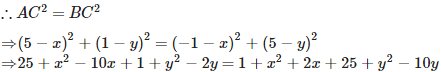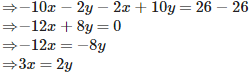Test: Coordinate Geometry- 5 - Question 2

### The points A(4, – 1), B(6, 0), C(7, 2) and D(5, 1) are the vertices of a

Detailed Solution for Test: Coordinate Geometry- 5 - Question 2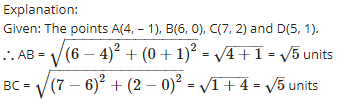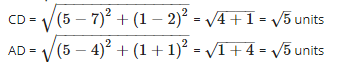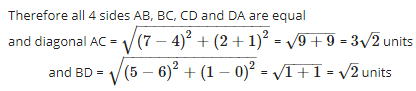Therefore diagonals AC and BD are not equal
Since, all sides are equal and both diagonals are not equal.
Therefore, the given quadrilateral is a rhombus.

Test: Coordinate Geometry- 5 - Question 3

### The co – ordinates of the mid – point of the line joining the points (3p, 4) and ( – 2, 4) are (5, p). The value of ‘p’ is

Detailed Solution for Test: Coordinate Geometry- 5 - Question 3

Explanation:

Let the coordinates of midpoint O(5,p) is equidistance from the points A(3p,4) and B(−2,4).( because O is the mid-point of AB)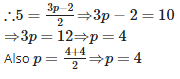Test: Coordinate Geometry- 5 - Question 4

If the points (2, 3), (4, k) and (6, – 3) are collinear, then the value of ‘k’ is

Detailed Solution for Test: Coordinate Geometry- 5 - Question 4

Explanation:

Let the points A (2, 3), B(4,k) and C(6,−3) be collinear.
If the points are collinear then area of triangle ABC formed by these three points is 0.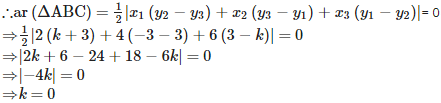Test: Coordinate Geometry- 5 - Question 5

The distance of a point from the y – axis is called

Detailed Solution for Test: Coordinate Geometry- 5 - Question 5

Explanation:

The distance of a point from the y – axis is the x (horizontal)  coordinate of the point and is  called abscissa.

Test: Coordinate Geometry- 5 - Question 6

If the point P(2, 4) lies on a circle, whose centre is C(5, 8), then the radius of the circle is

Detailed Solution for Test: Coordinate Geometry- 5 - Question 6

Explanation:The point P(2 , 4) is on the circle and C(5, 8) is its centre

Hence PC will be Radius of circle.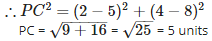Test: Coordinate Geometry- 5 - Question 7

The perimeter of a triangle with vertices (0, 4), (0, 0) and (3, 0) is

Detailed Solution for Test: Coordinate Geometry- 5 - Question 7

Explanation:

Given: the vertices of a triangle ABC, A(0, 4), B (0, 0) and C (3, 0).
∴ Perimeter of triangle ABC = AB + BC + AC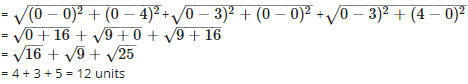Test: Coordinate Geometry- 5 - Question 8

The co – ordinates of the point which divides the join of ( – 6, 10) and (3, – 8) in the ratio 2 : 7 is

Detailed Solution for Test: Coordinate Geometry- 5 - Question 8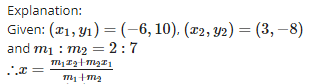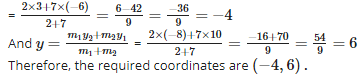Test: Coordinate Geometry- 5 - Question 9

If the points (x, y), (1, 2) and (7, 0) are collinear, then the relation between ‘x’ and ‘y’ is given by

Detailed Solution for Test: Coordinate Geometry- 5 - Question 9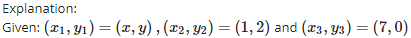and these are collinear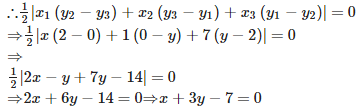Test: Coordinate Geometry- 5 - Question 10

The distance of a point from the x – axis is called

Detailed Solution for Test: Coordinate Geometry- 5 - Question 10

Explanation:The distance of a point from the x – axis is the y (vertical) coordinate of the point and is called ordinate.

Test: Coordinate Geometry- 5 - Question 11

A circle has its centre at the origin and a point P(5, 0) lies on it. Then the point Q(8, 6) lies ________ the circle.

Detailed Solution for Test: Coordinate Geometry- 5 - Question 11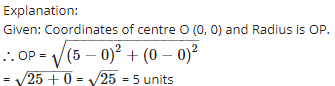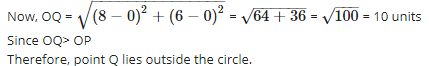Test: Coordinate Geometry- 5 - Question 12

(0, 3), (4, 0) and ( – 4, 0) are the vertices of

Detailed Solution for Test: Coordinate Geometry- 5 - Question 12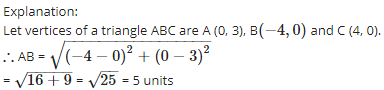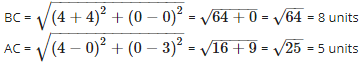Since, two sides are equal, therefore, ABC is an isosceles triangle.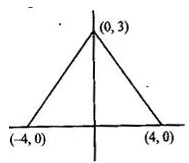Test: Coordinate Geometry- 5 - Question 13

The ratio in which the point (1, 3) divides the line segment joining the points ( – 1, 7) and (4, – 3) is

Detailed Solution for Test: Coordinate Geometry- 5 - Question 13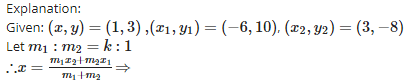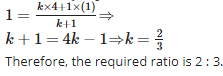Test: Coordinate Geometry- 5 - Question 14

If the vertices of a triangle are (1, 1), ( – 2, 7) and (3, – 3), then its area is

Detailed Solution for Test: Coordinate Geometry- 5 - Question 14

Explanation:

Given: (x1,y1)=(1,1),(x2,y2)=(−2,7) and (x3,y3)=(3,−3)=(3,−3), then the Area of trianlge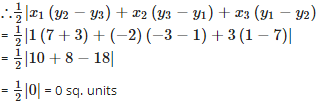Also therefore the three given points(vertices) are collinear

Test: Coordinate Geometry- 5 - Question 15

The point of intersection of the x – axis and y – axis is called

Detailed Solution for Test: Coordinate Geometry- 5 - Question 15

Explanation:The point of intersection of the x – axis and y – axis is called origin.The coordinates of origin are (0, 0).

## Quantitative Aptitude (Quant)

163 videos|163 docs|131 tests
 Use Code STAYHOME200 and get INR 200 additional OFF Use Coupon Code
Information about Test: Coordinate Geometry- 5 Page
In this test you can find the Exam questions for Test: Coordinate Geometry- 5 solved & explained in the simplest way possible. Besides giving Questions and answers for Test: Coordinate Geometry- 5, EduRev gives you an ample number of Online tests for practice

## Quantitative Aptitude (Quant)

163 videos|163 docs|131 tests

### How to Prepare for CAT

Read our guide to prepare for CAT which is created by Toppers & the best Teachers